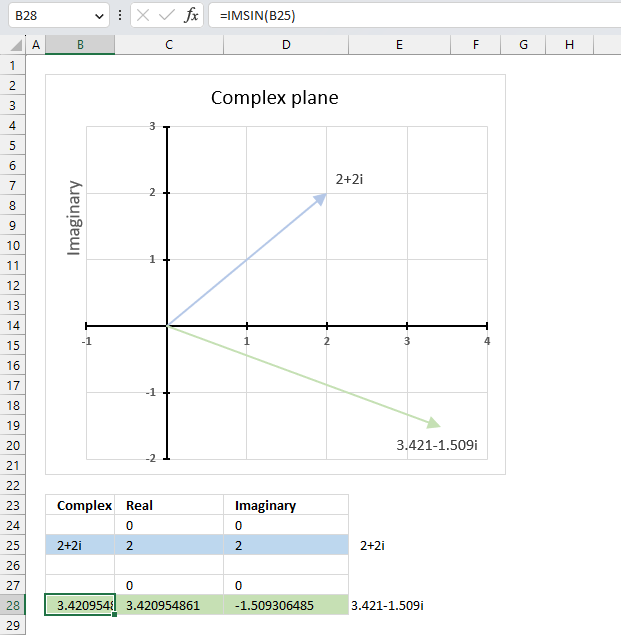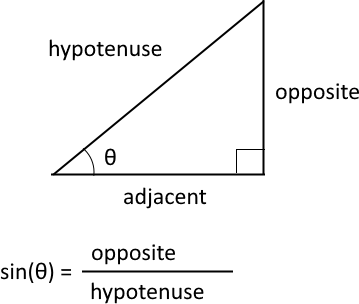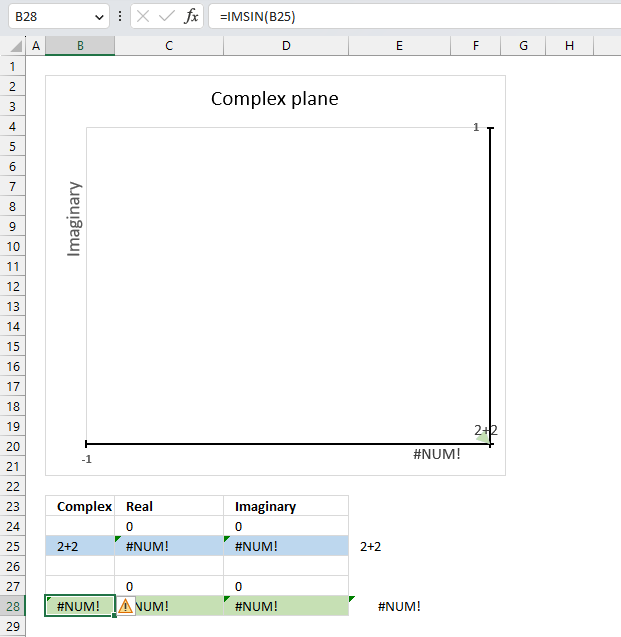Author: Oscar Cronquist Article last updated on June 02, 2023What is the IMSIN function?

The IMSIN function calculates the sine of a complex number in x + yi or x + yj text format.

The letter j is used in electrical engineering to distinguish between the imaginary value and the electric current.

What is the sine?The sine is a trigonometric function that relates an angle θ in a right triangle to the ratio of the length of the side opposite the angle and the length of the longest side (hypotenuse) of the triangle. A right triangle has one angle that measures 90° or π/2 radians which is approximately 1.5707963267949 radians.

What is the difference between sine and the complex sine?
The difference between sine and complex sine is that the former is defined for real numbers only, while the latter is defined for complex numbers as well. The sine of a complex number has some similarities to the sine of a real number, such as periodicity.

The sine function is periodic considering the angle, meaning it repeats its values after a certain interval. The period of the sine function is 2π or 360 degrees.

sin z = (e(iz) - e(-iz))/2i

z - complex number
i - imaginary unit
e -

IMSIN(inumber)

## 2. IMSIN Function Arguments

 inumber Required. A complex number in x+yi or x+yj text format.

## 3. IMSIN function exampleThe image above demonstrates a formula in cell B28 that calculates the sine of a complex number specified in cell B25.

Cell C28 calculates the real number from the complex number in cell B28. Cell D28 extracts the imaginary number from the complex number specified in cell B28.

The real and imaginary numbers separated in a cell each allow us to graph the complex number on the complex plane.

Formula in cell B28:

=IMSIN(B25)

The chart above shows the complex plane, the y-axis is the imaginary axis and the x-axis is the real axis.

Complex number 2+2i is the light blue line in the first quadrant. The sine of 2+2i is the green line shown in the fourth quadrant.

### 3.1 Explaining formula

IMSIN(inumber)

becomes

IMSIN(B25)

#### Step 2 - Evaluate the IMSIN function

IMSIN(B25)

becomes

IMSIN("2+2i")

and returns

3.42095486111701-1.50930648532362i

## 4. How to calculate the sine of a complex number in detail?The sine of a complex number is calculated like this:

C = x + yi

sin (C) = sin (x) cosh (y) + cos (x) sinh (y)i

For example, C=2+2i

sin (2+2i) = sin (2) cosh (2) + cos (2) sinh (2)i

becomes

sin (2+2i) = 0.909297426825682*3.76219569108363 + -0.416146836547142*3.62686040784702i

equals

sin (2+2i) = 3.42095486111701 - 1.50930648532361i

sin - calculates the sine of a number
cos - calculates the cosine of a number
cosh - calculates the hyperbolic cosine of a number
sinh - calculates the hyperbolic sine of a number

## 5. IMSIN function not workingThe IMSIN function returns a #NUM error if the provided argument is not a valid complex number.